Custom SearchCONSTANT TO A VARIABLE POWER In this section a discussion of two forms of a constant to a variable power is presented. The two forms are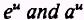, where u is a variable, a is any constant, and e is a defined constant. Formula.PROOF: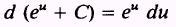Therefore,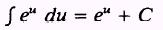EXAMPLE: Evaluate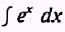SOL UTION: Letso thatThe integral is in the correct form to usetherefore, using substitution, we find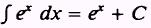EXAMPLE: Evaluate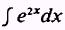SOL UTION: Let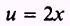so that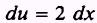We need a factor of 2 in the integral so that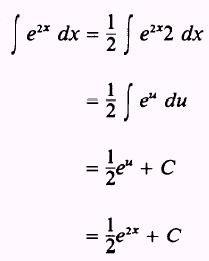EXAMPLE: Evaluate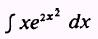SOL UTION: Let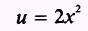so that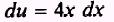Here a factor of 4 is needed in the integral; therefore,EXAMPLE: Evaluate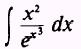SOLUTION: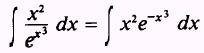Let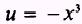so thatTherefore,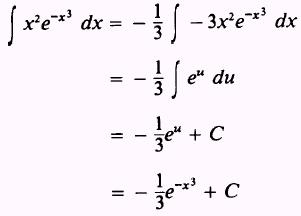PRACTICE PROBLEMS: Evaluate the following integrals: 1. J - 2xe x2 dxANSWERS:We will now discuss the second form of the integral of a constant to a variable power. Formula.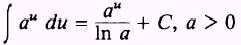Integrated Publishing, Inc. - A (SDVOSB) Service Disabled Veteran Owned Small Business